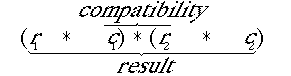Glossary of terms used in matrix algebra:
(bold: most important for us)

Compatibility:

Two matrices are compatible (for multiplication) if the number of columns in the first equals the number of rows in the second. This is because each row (with j elements) in the first matrix will be multiplied by each column (with i elements) in the second matrix. For the element by element principle to work, the number of elements (columns) in the first must equal the number of elements (rows) in the second. Heuristic: m x n * o x p leads to NO GO if n < > o. Do they match where they touch?
Compatibility: the "inside" terms  ( c1 must equal r2)Dimensionality of result : the "outside" terms   (r1 * c2)

Determinant (Det):

Square matrices only. Det A = · aij (-1)i+j Det Mij, where Mij is the minor of A with the ith row and jth column deleted. (Sum over the j's for expanding by columns, which is the normal way to do it). For larger matrices, calculating the determinant is done by row interchanges. The determinant can tell us about the number of solutions to a set of equations (unique, infinite?) and various other useful properties of the matrix.
Eigenvalues:
The characteristic roots of a set of simultaneous equations. In matrix form the eigenvalues (l) are defined such that Det(A - lI) = 0 [the characteristic equation]. The characteristic equation will be a polynomial in l of degree n, where n is the order (size) of the matrix. In demography, the dominant eigenvalue is the population growth rate, l, while the second eigenvalue gives the damping ratio (how quickly a population will return to equilibrium following a perturbation).
Eigenvector:
A vector, u, such that A*u = l*u.   For matrix models, the right and left eigenvectors corresponding to the dominant eigenvalue are the reproductuve values (left eigenvector, a row vector) and the stable (st)age distribution (right eigenvector, a column vector).
Identity matrix:
Matrix usually written as I, with 1 (ones) on the main diagonal and zeros elsewhere.
Inverse:
A-1 * A = I. The inverse of a matrix is that matrix which when premultiplied against A, yields the identity matrix. Inverse exists only when the matrix is singular (that is, has a determinant).
Irreducible:
Equivalent to "strongly connected" in diagraph theory (see Keyfitz p. 30). Each point on the graph (or in the matrix) can be reached from every other point, either directly or by going through intermediate nodes.
Leslie matrix:
Age-classified matrix used in life history analysis. It has elements only in the top row (fertility) and along the subdiagonal (survival). In order to produce a stable age distribution, the matrix must be irreducible (strongly connected) and primitive.
Multiplication:
see Compatibility. Done row by column, element by element. The product of r x c * c x s matrix is a matrix of order (= size) r x s (the number of rows in the first by the number of columns in the second). So, compatibility involves the inner two numbers and the 'order' of the product matrix involves the outer two numbers.
Primitive:
Any matrix for which An (for some arbitrary n) has only positive elements is said to be primitive. Primitivity of a nonnegative, irreducible matrix is a sufficient condition for stability. For the simple Leslie matrix this means that at least two ageclasses must exist that have mi > 0 and that have i relatively prime (e.g., for a six-year lifespan fecundity only at age-classes 3 and 6 will yield cycles rather than a stable age distribution).
Singular:
A matrix whose determinant is zero.

Subscript notation:

For a matrix A, aij means the element in the ith row and jth column. A = {aij}, where {} means "set of".
Symmetric:
A matrix is symmetric if it equals its own transpose. That is, if A + A'.
Trace:
Square matrices only. The sum of the elements of the main diagonal.
Transpose:
For a matrix A, the transpose A' = {arc}' = {acr} (use i and j as subscripts, if you prefer).  That is, the elements in the rows of the first become the elements of the columns of the transpose.
REFERENCES: Keyfitz, N. 1985. pp. 27-33 discusses matrices and graphs as used in demography.

Roughgarden, J. 1979. Appendix 3, pp. 579-590 In: Theory of Population Genetics and Evolutionary Ecology: An Introduction. MacMillan, N.Y.

Searle, S.R. 1966. Matrix Algebra for the Biological Sciences. Wiley and Sons, N.Y.